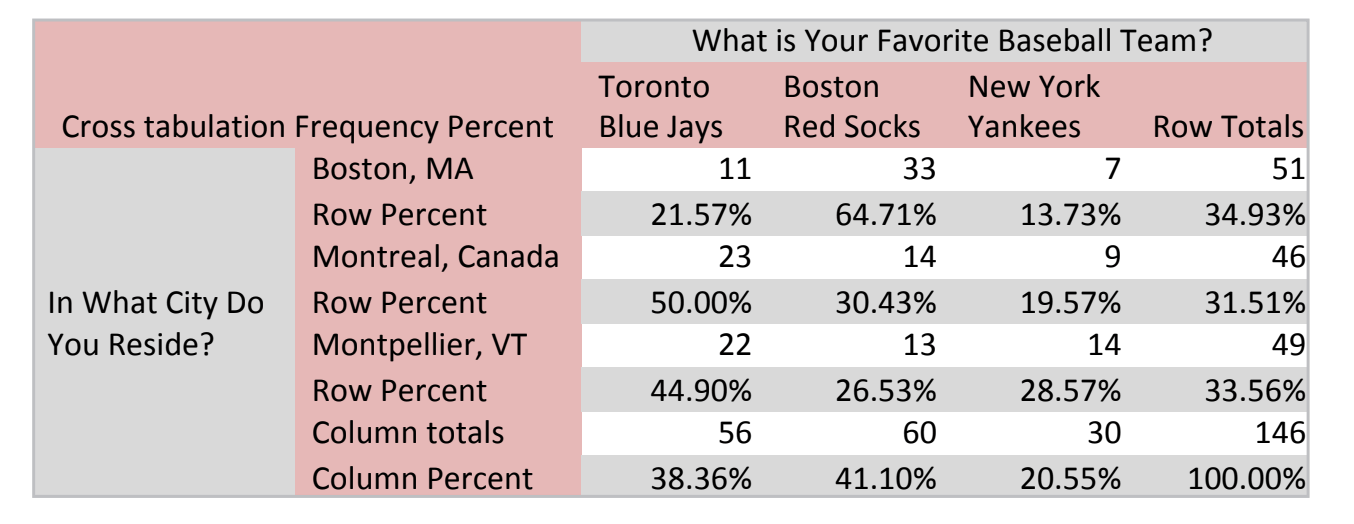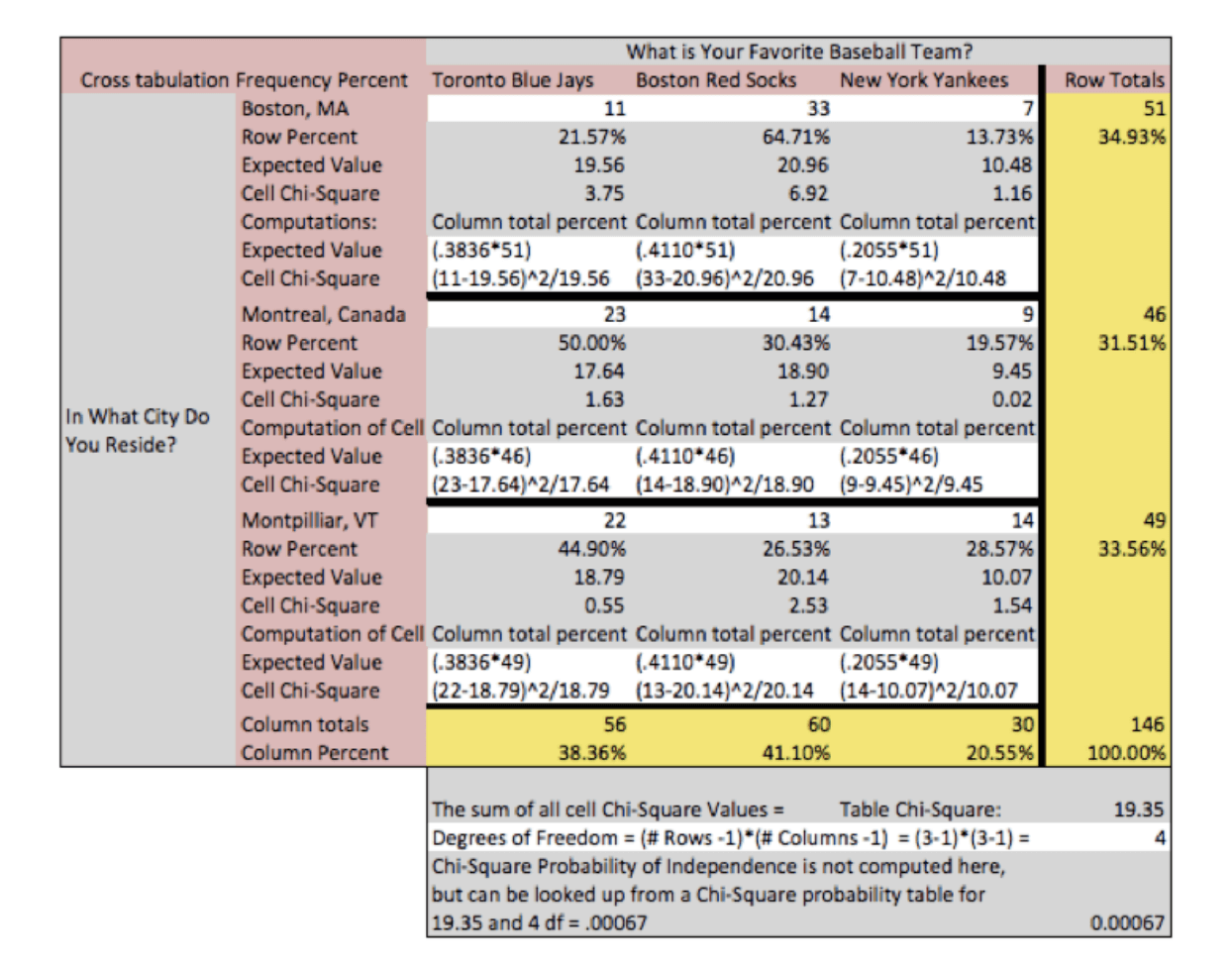# Cross-Tabulation Analysis: A Researchers Guide

6 min read
Cross-tabulation is one of the most useful analytical tools and a mainstay of the market research industry. Cross-tabulation analysis, also known as contingency table analysis, is most often used to analyze categorical (nominal measurement scale) data.

For reference, a cross-tabulation (or crosstab) is a two- (or more) dimensional table that records the number (frequency) of respondents that have the specific characteristics described in the cells of the table. Cross-tabulation tables provide a wealth of information about the relationship between the variables. Cross-tabulation analysis goes by several names in the research world including crosstab, contingency table, chi-square and data tabulation.

Cross-tabulation analysis has its own unique language, using terms such as “banners”, “stubs”, “Chi-Square Statistic” and “Expected Values.” A typical cross-tabulation table comparing the two hypothetical variables “City of Residence” with “Favorite Baseball Team” is shown below. Are city of residence and being a fan of that team independent? The cells of the table report the frequency counts and percentages for the number of respondents in each cell.

See how Qualtrics makes Cross Tabs simpleIn this table, the text legend in the crosstab describes the row and column variables. You can create and analyze multiple tables in a side-by-side or sequential format. Tabulation professionals call the column variables in these multiple tables “Banners” and row variables “Stubs”.

## Cross-Tabulation With Chi-Square Analysis

The Chi-square statistic is the primary statistic used for testing the statistical significance of the cross-tabulation table. Chi-square tests determine whether or not the two variables are independent. If the variables are independent (have no relationship), then the results of the statistical test will be “non-significant” and we are not able to reject the null hypothesis, meaning that we believe there is no relationship between the variables. If the variables are related, then the results of the statistical test will be “statistically significant” and we are able to reject the null hypothesis, meaning that we can state that there is some relationship between the variables.

The chi-square statistic, along with the associated probability of chance observation, may be computed for any table. If the variables are related (i.e., the observed table relationships would occur with very low probability, say only 5%) then we say that the results are “statistically significant” at the .05 or 5% level.

This means that the variables have a low chance of being independent. Students of statistics will recall that the probability values (.05 or .01) reflect the researcher’s willingness to accept a type I error, or the probability of rejecting a true null hypothesis (meaning that we thought there was a relationship between the variables when there really wasn’t).

Furthermore, these probabilities are cumulative, meaning that if 20 tables are tested, the researcher can be almost assured that one of the tables is incorrectly found to have a relationship (20 x .05 = 100% chance). Depending on the cost of making mistakes, the researcher may apply more stringent criteria for declaring significance, such as .01 or .005.

## Computation of the Chi-Square Statistic for Cross-Tabulation Tables

The chi-square statistic is computed by first computing a chi-square value for each individual cell of the table and then summing them up to form a total chi-square value for the table. The chi-square value for the cell is computed as: (Observed Value – Expected Value)2 / (Expected Value). The chi-Square computations are highlighted in gray.

In this example table, we observe that the chi-square value for the table is 19.35, and has an associated probability of occurring by chance less than one time in 1000. We therefore reject the null hypothesis of no difference and conclude that there must be a relationship between the variables. We can observe the relationship in two places in the table.

The most obvious is in the chi-square value computed for each cell. We observe that the cells “Red Socks and Boston”, “Blue Jays and Montreal” and “Red Socks and Montpellier, Vermont” were the three cells where the number of observed respondents was greater than expected. We further note that when we examine the expected and observed frequencies, the “Yankees and Montreal”, “Red Socks and Montpellier, Vermont”, and “Red Socks and Montreal” frequencies were fewer than expected.Because the cell chi-square and the expected values are often not displayed, these same relationships can be observed by comparing the column total percent to the cell percent (of the row total). In cell “Red Socks and Boston” we would compare 41.10% with 64.71% and observe that more Red Socks fans liked Boston than expected. Caution is urged when interpreting relationships found in any statistical analysis. We often desire to “explain” or conclude “causality” from analyses when data either is not designed to, or does not have the power to support such conclusions.

In the current table, we observe that “Red Socks and Boston” had the greatest delta between the number of observed and expected respondents, for any team preference and city of residence. However, we must be careful in concluding that the Red Socks caused respondents to move to Boston, or that Boston as a city of residence causes fan loyalty. Red Socks and Boston are the most observed fan and city relationship but are most likely totally independent when considering other concepts or relationships.

Crosstabs and chi-square are powerful ways to analyze your survey data. Another popular tool that makes an impact on research is Conjoint Analysis.

eBook: 8 innovations to modernize market research